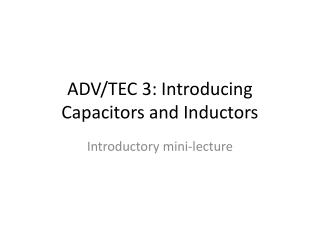Download PresentationADV/TEC 3: Introducing Capacitors and Inductors

# ADV/TEC 3: Introducing Capacitors and Inductors

Download Presentation## ADV/TEC 3: Introducing Capacitors and Inductors

- - - - - - - - - - - - - - - - - - - - - - - - - - - E N D - - - - - - - - - - - - - - - - - - - - - - - - - - -
##### Presentation Transcript

1. ADV/TEC 3: Introducing Capacitors and Inductors Introductory mini-lecture

2. Capacitors • A capacitor is any two conductors separated by an insulator • Voltage across a capacitor is proportional to the charge on each plate, q = Cv

3. Capacitors • Apply voltage v = V0ejωt • Charge builds up on plates, q = C V0 ejωt • A change in voltage drives electrons from one plate to the other around the circuit, producing a current • j indicates a 90o phase difference between i & v i.e., v ‘lags’ i by 90o (or π/2) • Impedance Z = v/i= 1/jωC, Reactance XC = -1/ωC